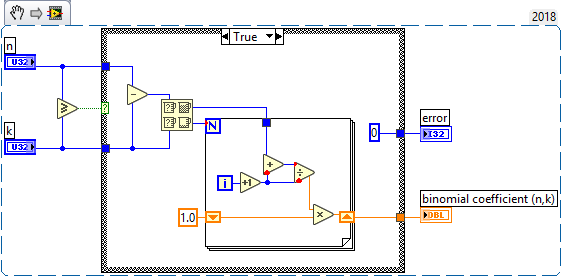# LabVIEW

cancel
Showing results for
Did you mean:

## Re: enumerate combinations

215 pick 2 = (215*214)/(2) = 23005

215 pick 3 = (215*214*213)/(3*2) =  1633355

215 pick 4 = (215*214*213*212)/(4*3*2) = 86567815

636 pick 6 = (636*635*634*633*632*631)/(6*5*4*3*2) = 89771348696212

and so on....

or am I missing something.

Message 21 of 33
(908 Views)

## Re: enumerate combinations

@Intaris or am I missing something.

No, you are not missing anything.

I thought the OP wanted a list of all combinations, ie, something like below for 215 pick 2, not just the size of possible combinationsmcduff

Message 22 of 33
(883 Views)

## Re: enumerate combinations

Well, if n and r are "not so small", the problem begins to get out of hand.  How many Bridge Hands are there?  That is, what is 52 choose 13?  Easy to write down (knowing the Binomial Formula, n!/((n-r)!r!) as

(52 * 51 * 50 * 49 * 48 * 47 * 46 * 45 * 44 * 43 * 42 * 41 * 40) / 13!

(I'm too lazy to write out 13!).  How big is the numerator?  Let's do a rough "back-of-the-envelope" and say it is on-the-order-of 50^13 = 100^13/2^13 ~ 10^26/10^4 = 10^22.  If we now say 10^3 ~ 2^10 (I'm using ~ to mean "close enough for this discussion"), this is on the order of 70 bits, which is bigger than an I64 and more than the 53 bits of precision in Double Precision.

How about Extended Precision?  Well, the "precision" is a few bits more, namely 65, still not enough!

Indeed, the correct answer (according to Mathematica) is 635013559600, approximately a 40 bit number, so you'd need to use I64 (or Dbl) to compute it.

Bob Schor

Message 23 of 33
(870 Views)

## Re: enumerate combinations

Well, it turns out that LabVIEW also gets the "right" answer.  How?  It basically does the division at the same time as it does the multiplication, so there are as many divisions (keeping the numbers being multiplied smaller) as there are multiplications.  I'm not sure how it manages to avoid "unfortunate" rounding errors when doing the division -- oh, they can probably guarantee an "exact" division by ordering the terms correctly (have the Numerator go from Large to Small, and the Denominator from Small to Large).

Bob Schor

Message 24 of 33
(865 Views)

## Re: enumerate combinations

@Bob_Schor wrote:

Well, it turns out that LabVIEW also gets the "right" answer.  How?  It basically does the division at the same time as it does the multiplication, so there are as many divisions (keeping the numbers being multiplied smaller) as there are multiplications.  I'm not sure how it manages to avoid "unfortunate" rounding errors when doing the division -- oh, they can probably guarantee an "exact" division by ordering the terms correctly (have the Numerator go from Large to Small, and the Denominator from Small to Large).

Bob Schor

Well lets not guessThere are a few things I don't understand here like:

1. since n and k are positive And k is not > n, WHAT is the Min Max doing there?
2. What happens when n-k>2147483647? Thats a lot of code to generate "1"
3. What happens when n-k=2147483647? (the last iteration will have a negative denominator won't it?)
4. Where's the absval for the case 3 bug?
5. IMHO A Compound arithmetic node would make it a bit cleaner.

Another candidate for NI to lock the BD...

"Should be" isn't "Is" -Jay
Message 25 of 33
(857 Views)

## Re: enumerate combinations

WHAT is the Min Max doing there?

n - k or in other words n minus k goes to Min and Max versus k., Not Max and Min of n and k

So I can answer that question.

mcduff

Message 26 of 33
(854 Views)

## Re: enumerate combinations

@mcduff wrote:

WHAT is the Min Max doing there?

n - k or in other words n minus k goes to Min and Max versus k., Not Max and Min of n and k

So I can answer that question.

mcduff

Show me numbers that can get to that case where n-k>n  we would execute the false case and throw error -23045

"Should be" isn't "Is" -Jay
Message 27 of 33
(851 Views)

## Re: enumerate combinations

Show me numbers that can get to that case where n-k>n  we would execute the false case and throw error -23045

There aren't any.

The 1st comparison checks if the numbers are kosher, n needs to be greater than or equal to k.

The min/max determines how many times the for loop runs, assume n =3 k=2, n-k =1

Then the loop would run 1 time, now

assume n =5 k=2, n-k =3, the loop would run 2 times.

The min are different in these cases once it is n-k, the other it's k

Message 28 of 33
(847 Views)

## Re: enumerate combinations

@mcduff wrote:

Whoops

"Should be" isn't "Is" -Jay
Message 29 of 33
(842 Views)

## Re: enumerate combinations

The Max/Min is that you are comparing the two parts of the denominator, n-k and k.  You want to "cancel terms" with the maximum number of terms.

Bob Schor

Message 30 of 33
(840 Views)